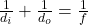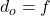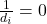Question

At the focal point of a converging lens, the object:
A. appears inverted
B. can be seen normally
C. appears reversed (left/right)
D. cannot be seen

1.D. cannot be seen

Explanation:

2.D) Image cannot be seen

Explanation:

As we know by lens formulanow we know that

object is placed at the focal point of lensso we will haveso the image will form at infinite

so image will be at infinite distance from the lens

so it cannot be seen